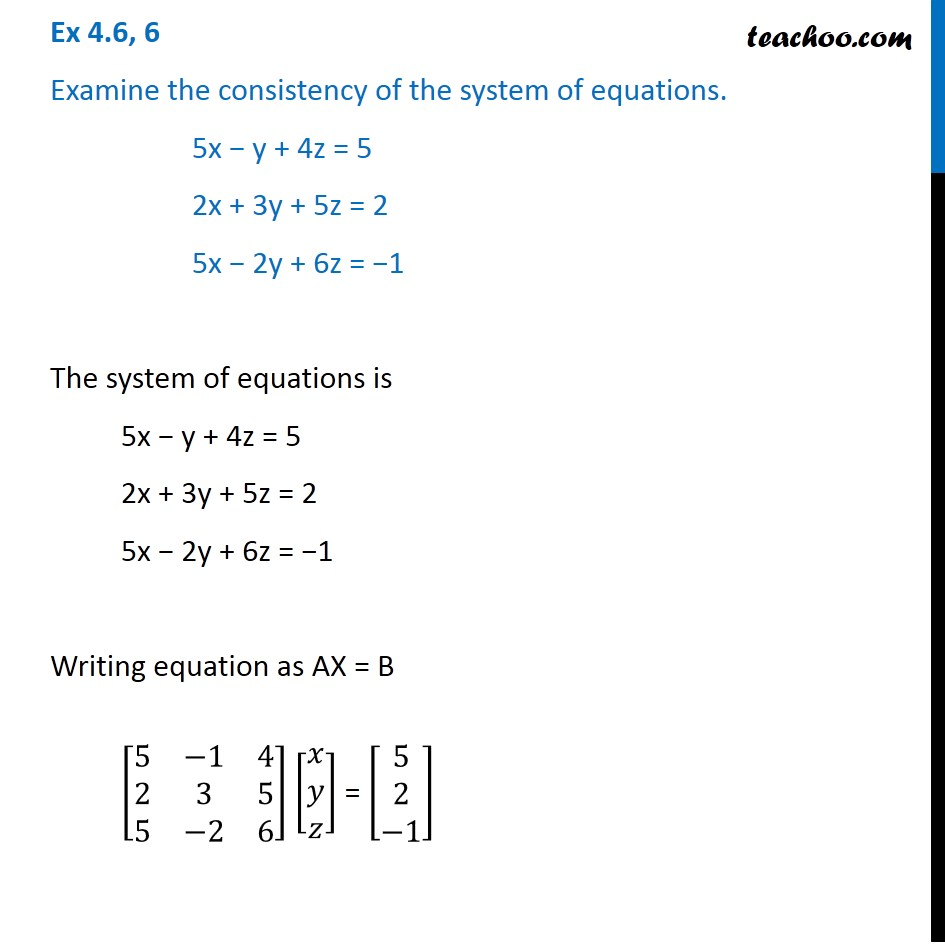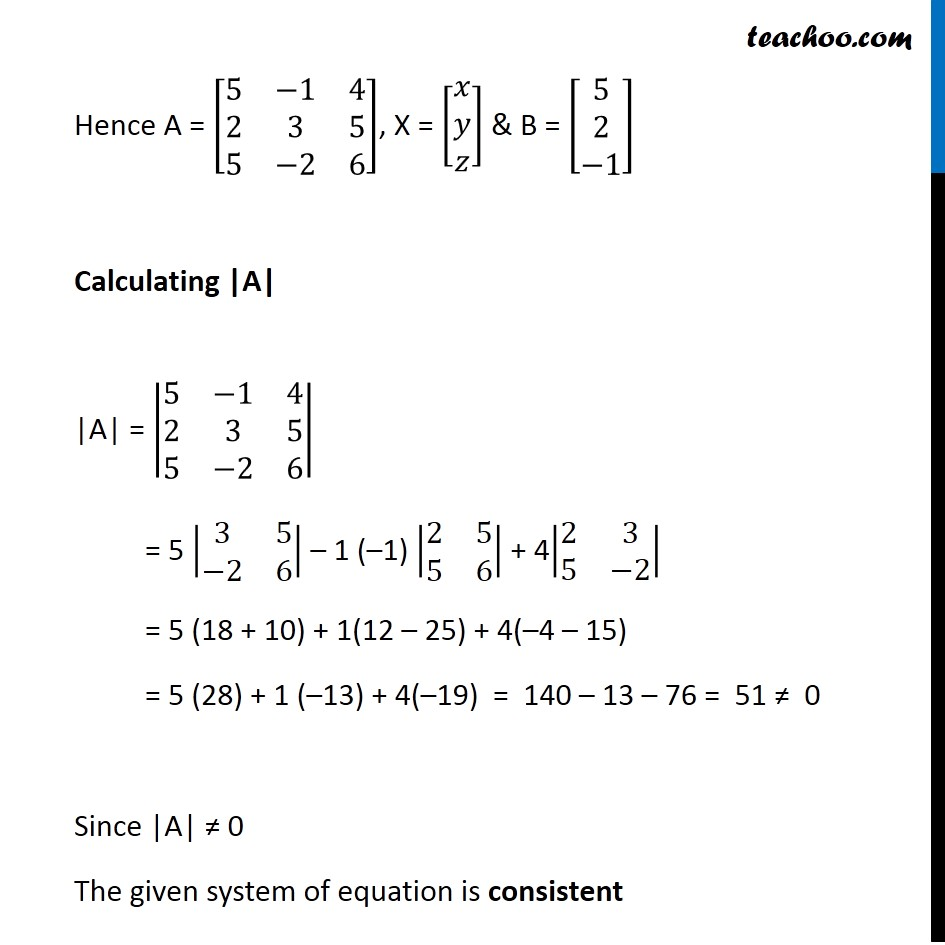Ex 4.6

Chapter 4 Class 12 Determinants (Term 1)
Serial order wise### Transcript

Ex 4.6, 6 Examine the consistency of the system of equations. 5x − y + 4z = 5 2x + 3y + 5z = 2 5x − 2y + 6z = −1 The system of equations is 5x − y + 4z = 5 2x + 3y + 5z = 2 5x − 2y + 6z = −1 Writing equation as AX = B [■8(5&−1&4@2&3&5@5&−2&6)] [■8(𝑥@𝑦@𝑧)] = [■8(5@2@−1)] Hence A = [■8(5&−1&4@2&3&5@5&−2&6)], X = [■8(𝑥@𝑦@𝑧)] & B = [■8(5@2@−1)] Calculating |A| |A| = |■8(5&−1&4@2&3&5@5&−2&6)| = 5 |■8(3&5@−2&6)| – 1 (–1) |■8(2&5@5&6)| + 4|■8(2&3@5&−2)| = 5 (18 + 10) + 1(12 – 25) + 4(–4 – 15) = 5 (28) + 1 (–13) + 4(–19) = 140 – 13 – 76 = 51 ≠ 0 Since |A| ≠ 0 The given system of equation is consistent